# Question 1 1. [5 pts] Give a complete definition of lim f(x) = -oo if... 2....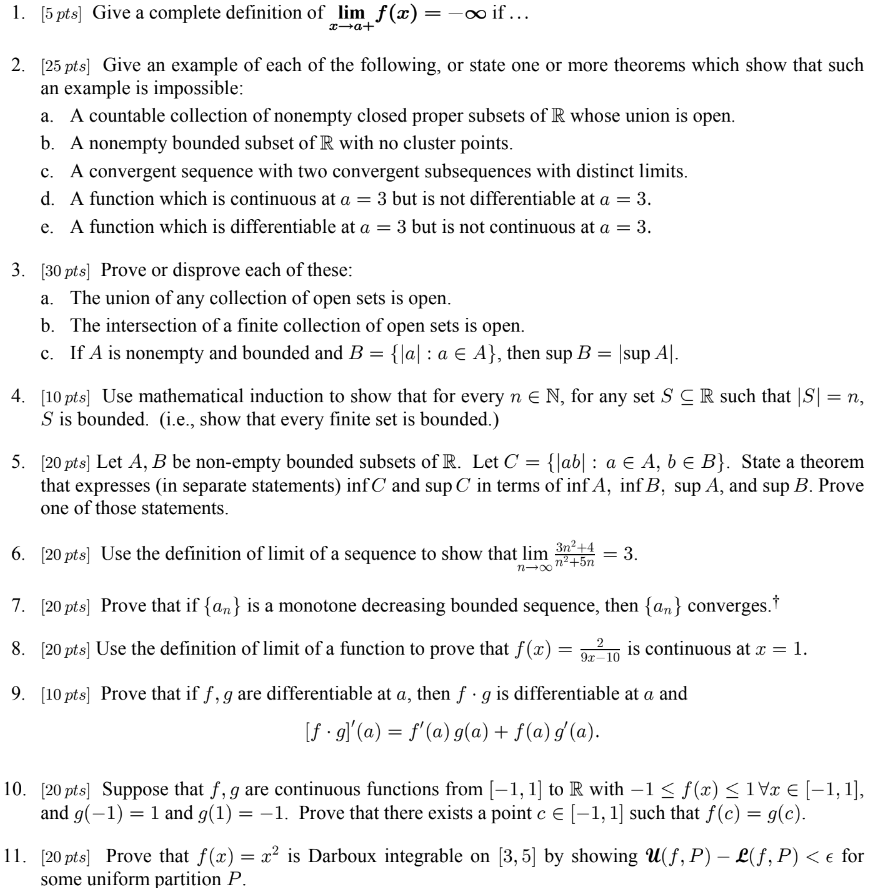Question 1

1. [5 pts] Give a complete definition of lim f(x) = -oo if... 2. [25 pts] Give an example of each of the following, or state one or more theorems which show that such an example is impossible: a. A countable collection of nonempty closed proper subsets of R whose union is open. b. A nonempty bounded subset of R with no cluster points. c. A convergent sequence with two convergent subsequences with distinct limits. d. A function which is continuous at a = 3 but is not differentiable at a = 3. e. A function which is differentiable at a = 3 but is not continuous at a = 3. 3. [30 pts] Prove or disprove each of these: a. The union of any collection of open sets is open. b. The intersection of a finite collection of open sets is open. c. If A is nonempty and bounded and B = {\al: a € A}, then sup B = |sup Al. 4. [10 pts] Use mathematical induction to show that for every n e N, for any set SCR such that |SI = n, S is bounded. (i.e., show that every finite set is bounded.) 5. (20 pts] Let A, B be non-empty bounded subsets of R. Let C = {[ab] : a € A, B E B}. State a theorem that expresses (in separate statements) infC and sup C in terms of inf A, inf B, sup A, and sup B. Prove one of those statements. 6. (20 pts] Use the definition of limit of a sequence to show that lim 3n2+4 3. n- na+5n 7. (20 pts] Prove that if {an} is a monotone decreasing bounded sequence, then {an} converges. 8. (20 pts] Use the definition of limit of a function to prove that f(x) is continuous at x = 1. 9.C-10 9. [10 pts] Prove that if f, g are differentiable at a, then f .g is differentiable at a and [f.g)'(a) = f'(a)g(a) + f(a)g(a). 10. [20 pts] Suppose that f, g are continuous functions from 1-1, 1) to R with -1 <f(x) <1Vx € (-1,1], and g(-1) = 1 and g(1) = -1. Prove that there exists a point c € (-1, 1) such that f(0) = g(c). 11. (20 pts] Prove that f(x) = x2 is Darboux integrable on [3, 5] by showing U(f,P) - L(f,P) < e for some uniform partition P.

This Homework Help Question: "Question 1 1. [5 pts] Give a complete definition of lim f(x) = -oo if... 2...." No answers yet.

We need 10 more requests to produce the answer to this homework help question. Share with your friends to get the answer faster!

0 /10 have requested the answer to this homework help question.

Once 10 people have made a request, the answer to this question will be available in 1-2 days.
All students who have requested the answer will be notified once they are available.

#### Earn Coin

Coins can be redeemed for fabulous gifts.

Similar Homework Help Questions
• ### 2. If S:= {1/n - 1/min, me N}, find inf S and sup S. 4. Let...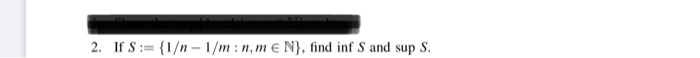2. If S:= {1/n - 1/min, me N}, find inf S and sup S. 4. Let S be a nonempty bounded set in R. (a) Let a > 0, and let aS := {as : S ES). Prove that inf(as) = a infs, sup(as) = a sup S. (b) Let b <0 and let b = {bs : S € S}. Prove that inf(bs) = b supS, sup(bs) = b inf S. 6. Let X be a nonempty set and...

• ### (5) Let f: [0, 1 R. We say that f is Hölder continuous of order a e (0,1) if \f(x) -- f(y)| . , y sup [0, 1] with 2 # 1...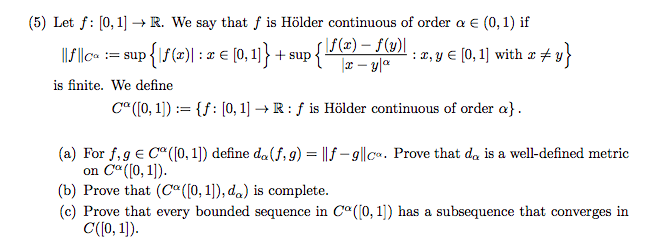(5) Let f: [0, 1 R. We say that f is Hölder continuous of order a e (0,1) if \f(x) -- f(y)| . , y sup [0, 1] with 2 # 1£l\c° sup is finite. We define Co ((0, 1]) f: [0, 1] -R: f is Hölder continuous of order a}. = (a) For f,gE C ([0, 1]) define da(f,g) = ||f-9||c«. Prove that da is a well-defined metric Ca((0, 1) (b) Prove that (C ([0, 1]), da) is complete...

• ### 5a) (5 pts) Find lim inf (xn) and lim sup (rn), for rn = 4 +...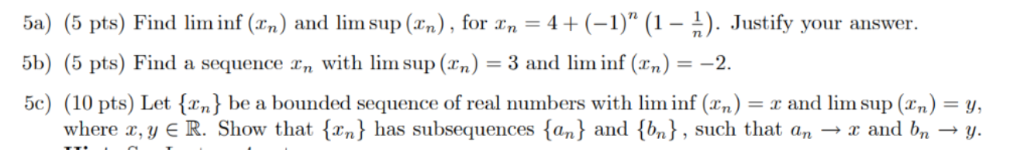5a) (5 pts) Find lim inf (xn) and lim sup (rn), for rn = 4 + (-1)" (1 - 2). Justify your answer 5b) (5 pts) Find a sequence r, with lim sup (xn) = 3 and lim inf (x,) = -2. 5c) (10 pts) Let {x,} be a bounded sequence of real numbers with lim inf (x,) = x and lim sup (x,) = y where , yER. Show that {xn} has subsequences {an} and {bn}, such that an...

• ### 5- Recall that a set KCR is said to be compact if every open cover for...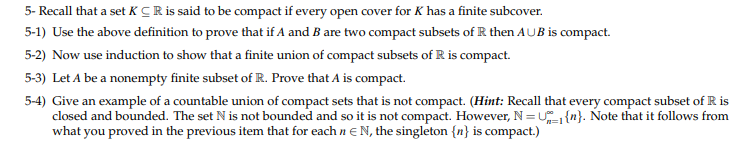5- Recall that a set KCR is said to be compact if every open cover for K has a finite subcover 5-1) Use the above definition to prove that if A and B are two compact subsets of R then AUB is compact induction to show that a finite union of compact subsets of R is compact. 5-2) Now use 5-3) Let A be a nonempty finite subset of R. Prove that A is compact 5-4) Give an example of...

• ### PLEASE ANSWER ALL! SHOWS STEPS 2. (a) Prove by using the definition of convergence only, without using limit theo- (b) Prove by using the definition of continuity, or by using the є_ó property, that 3...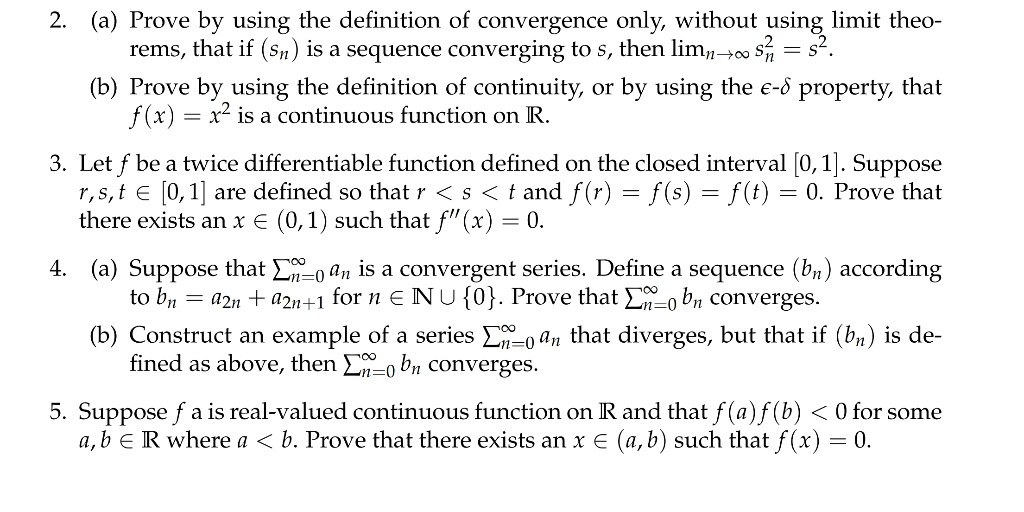PLEASE ANSWER ALL! SHOWS STEPS 2. (a) Prove by using the definition of convergence only, without using limit theo- (b) Prove by using the definition of continuity, or by using the є_ó property, that 3. Let f be a twice differentiable function defined on the closed interval [0, 1]. Suppose rems, that if (S) is a sequence converging to s, then lim, 10 2 f (x) is a continuous function on R r,s,t e [0,1] are defined so that r...

• ### 4. (20 pts) Let {xn} be a Cauchy sequence. Show that a) (5 pts) {xn} is...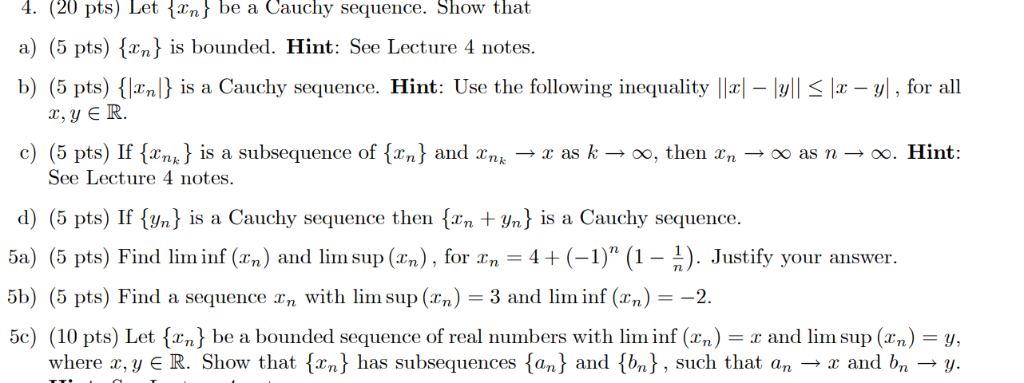4. (20 pts) Let {xn} be a Cauchy sequence. Show that a) (5 pts) {xn} is bounded. Hint: See Lecture 4 notes b) (5 pts) {Jxn} is a Cauchy sequence. Hint: Use the following inequality ||x| - |y|| < |x - y|, for all x, y E R. _ subsequence of {xn} and xn c) (5 pts) If {xnk} is a See Lecture 4 notes. as k - oo, then xn OO as n»oo. Hint: > d) (5 pts) If...

• ### For Topology!!! Match the terms and phrases below with their definitions. X and Y represents topological spaces. Note: there are more terms than definitions! Terms: compact, connected, Hausdorff, hom...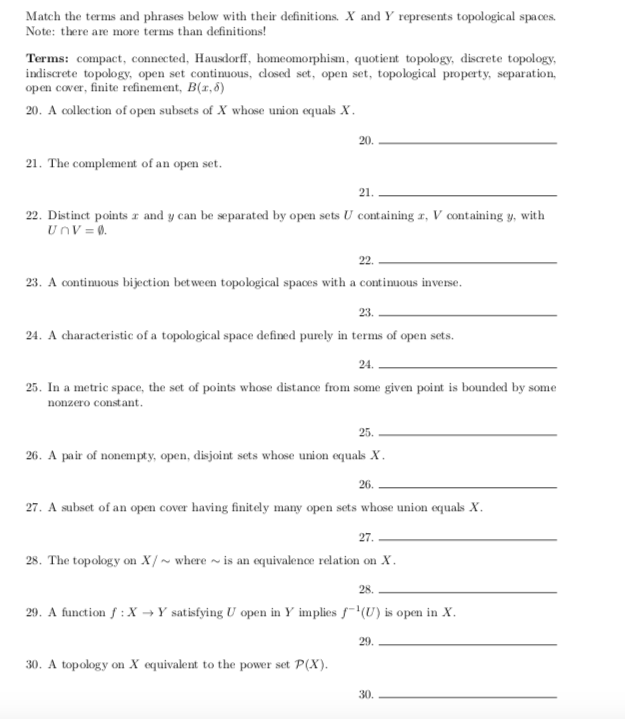For Topology!!! Match the terms and phrases below with their definitions. X and Y represents topological spaces. Note: there are more terms than definitions! Terms: compact, connected, Hausdorff, homeomorphis, quotient topology, discrete topology, indiscrete topology, open set continuous, closed set, open set, topological property, separation, open cover, finite refinement, B(1,8) 20. A collection of open subsets of X whose union equals X 20. 21. The complement of an open set 21. 22. Distinct points r and y can be separated...

• ### B2. (a) Let I denote the interval 0,1 and let C denote the space of continuous functions I-R. Def...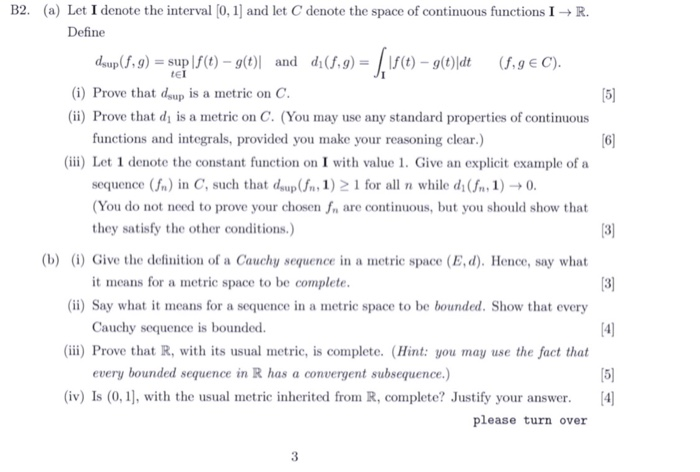B2. (a) Let I denote the interval 0,1 and let C denote the space of continuous functions I-R. Define dsup(f,g)-sup |f(t)-g(t) and di(f.g)f (t)- g(t)ldt (f,g E C) tEI (i) Prove that dsup is a metric on C (ii) Prove that di is a metric on C. (You may use any standard properties of continuous functions and integrals, provided you make your reasoning clear.) 6 i) Let 1 denote the constant function on I with value 1. Give an explicit...

• ### Write ‘T' for true or ‘F' for false. You do not need to show any work...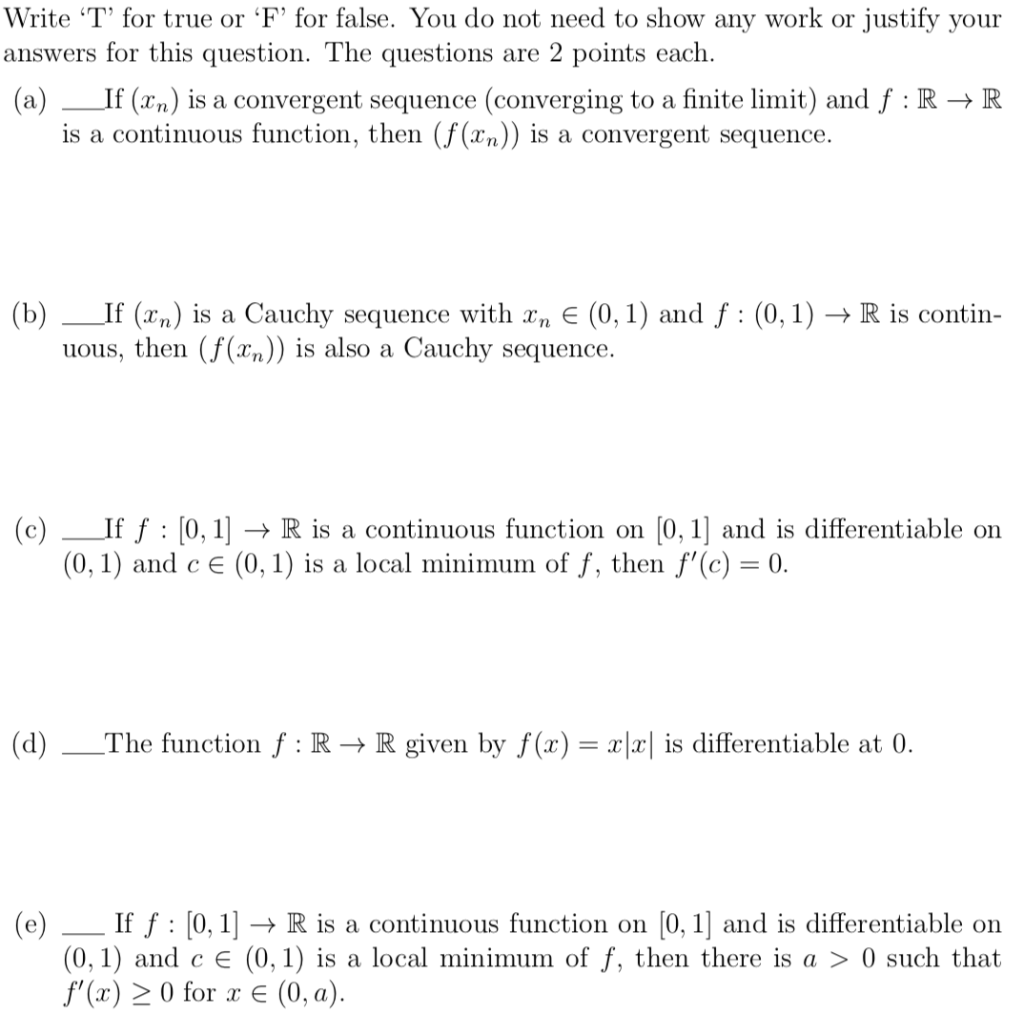Write ‘T' for true or ‘F' for false. You do not need to show any work or justify your answers for this question. The questions are 2 points each. (a) __If (xn) is a convergent sequence (converging to a finite limit) and f:RR is a continuous function, then (f (xn)) is a convergent sequence. (b) _If (xn) is a Cauchy sequence with Yn € (0,1) and f :(0,1) + R is contin- uous, then (f(xn)) is also a Cauchy sequence....

• ### Part 2: Metrics and Norms 1. Norms and convergence: (a) Prove the l2 metric defined in...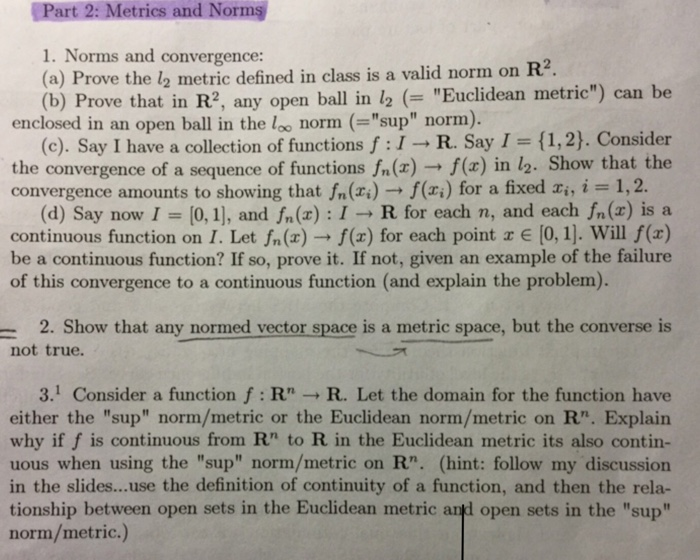Part 2: Metrics and Norms 1. Norms and convergence: (a) Prove the l2 metric defined in class is a valid norm on R2 (b) Prove that in R2, any open ball in 12 ("Euclidean metric") can be enclosed in an open ball in the loo norm ("sup" norm). (c). Say I have a collection of functions f:I R. Say I (1,2). Consider the convergence of a sequence of functions fn (z) → f(x) in 12-Show that the convergence amounts to...